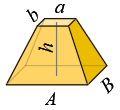### Volume and Surface Area of Rectangular Frustum (Obelisk)Dimensions

 Bottom base - A = B = Top base - a = b = Height - h =

Heights of side faces

ha =  h2 + (Bb)24

hb =  h2 + (Aa)24

Volume

V = h6·(A·b + a·B + 2·(a·b + A·B))

Area of side faces

So = ha·(A + a) + hb·(B + b)

Base areas

A1 = A·B

A2 = a·b

Total surface area

S = So + A1 + A2